# Relativity and Black Holes Black Holes

Next, let's solve the field equations for a spherically symmetric nonrotating mass M at rest at the origin of a coordinate system. The solution to this boundary value problem will tell us everything we need to know about the dynamics of a nonrotating, uncharged black hole.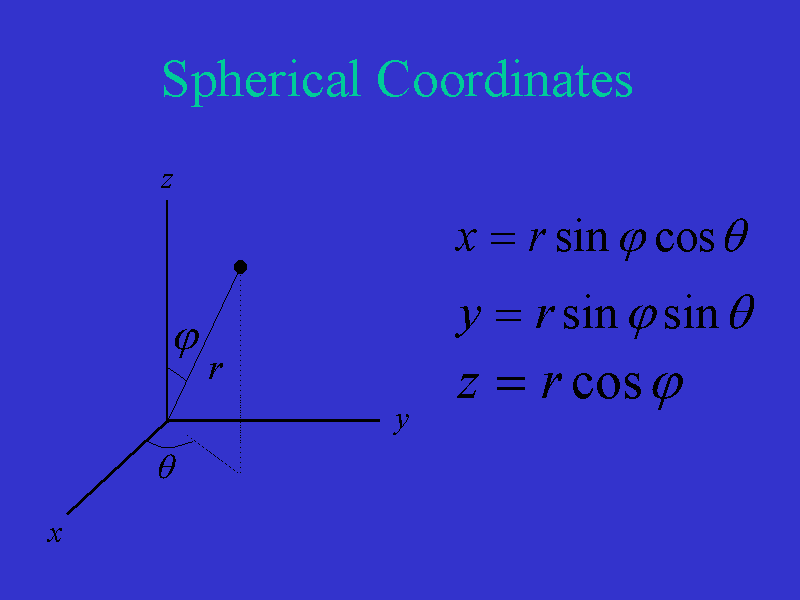Due to the high level of symmetry with which we are dealing, we will formulate the current problem in spherical coordinates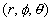. Here we see the relationships between rectangular coordinates (x,y,z) and spherical coordinates. The relationship between rectangular and spherical coordinates are as given.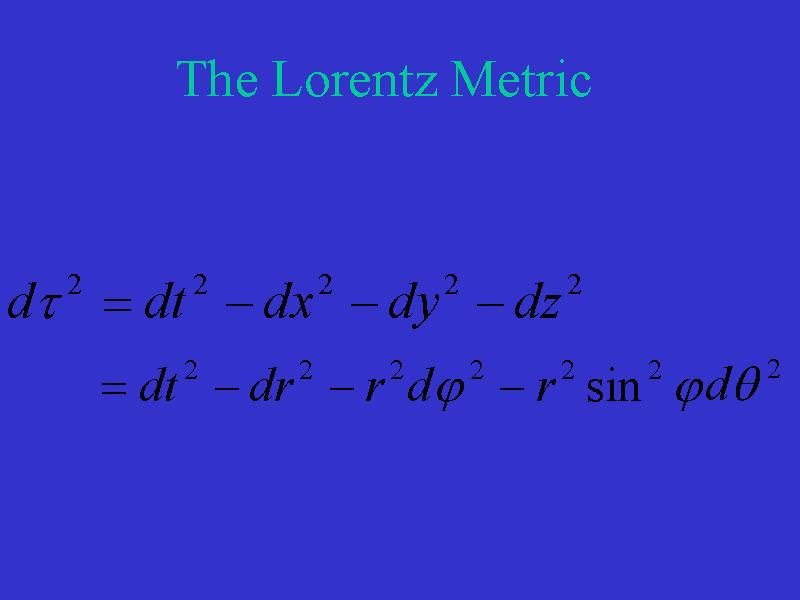In flat spacetime, the Lorentz metric in spherical coordinates is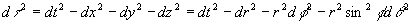We can verify this simply by computing the differentials of dt, dx, dy, dz and substituting into the expression for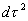as expressed in rectangular coordinates.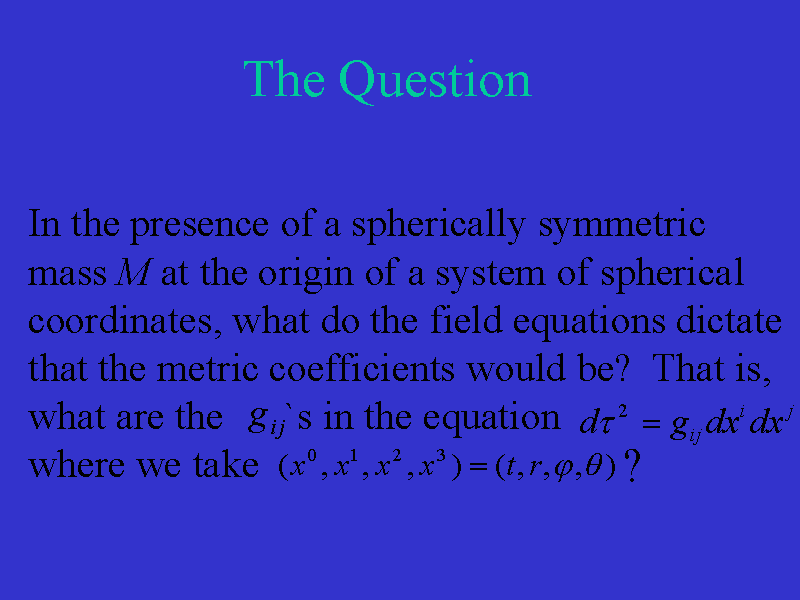Explicitly, the question we are addressing is this: "In the presence of a spherically symmetric mass M at the origin of a system of spherical coordinates, what do the field equations dictate that the metric coefficients would be? That is, what are the gij's in the equation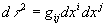where we take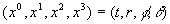." Notice that we have assumed a static situation. That is, a time independent situation.Therefore, our values of the gij's will only depend on r and M. In 1916, Karl Schwarzschild presented the solution to this problem. His solution is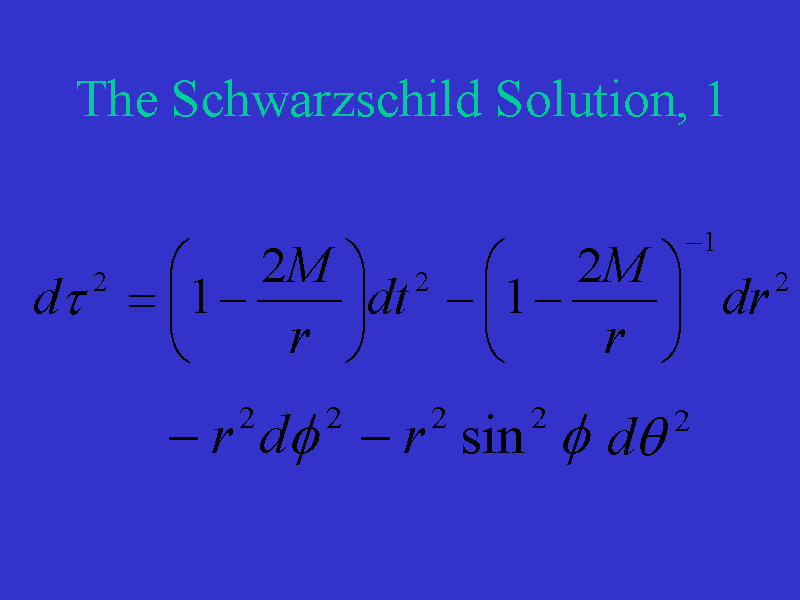More explicitly, Schwarzschild's solution to the field equations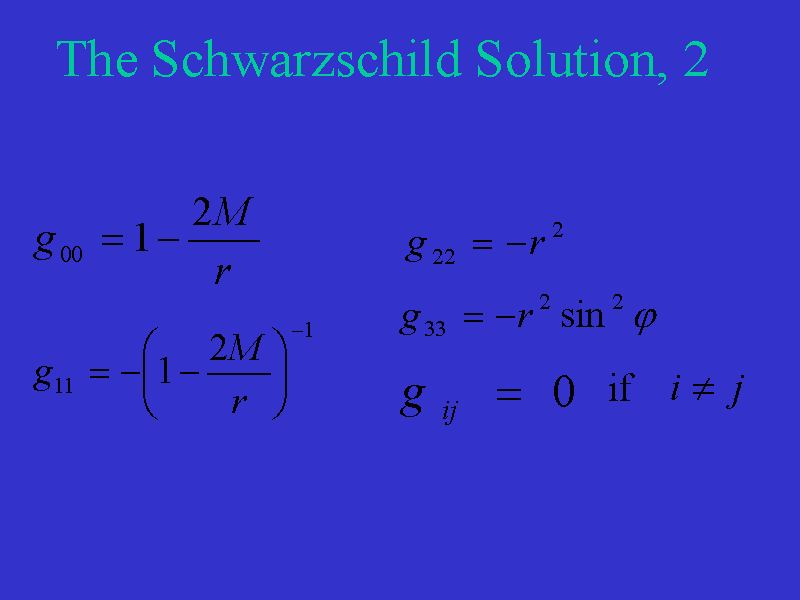in terms of the metric coefficients is as seen here. The field equations as stated earlier are only valid outside of the relevant massive object.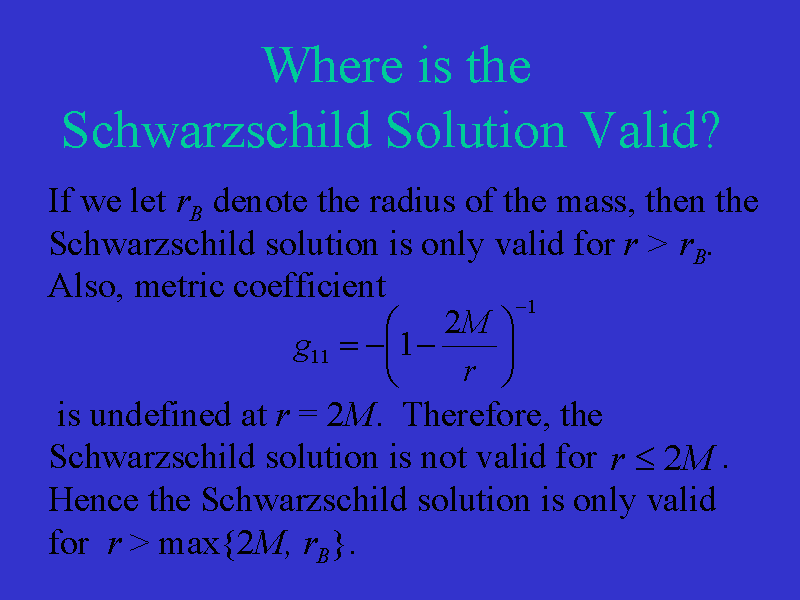If we let rB denote the radius of the spherically symmetric mass, then the Schwarzschild solution is only valid for r > rB. Also, we see that the metric coefficient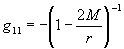is undefined at r = 2M (remember that we are using geometric units here). Therefore, the Schwarzschild solution is not valid for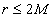. Hence the Schwarzschild solution is only valid for r > max{2M,rB}.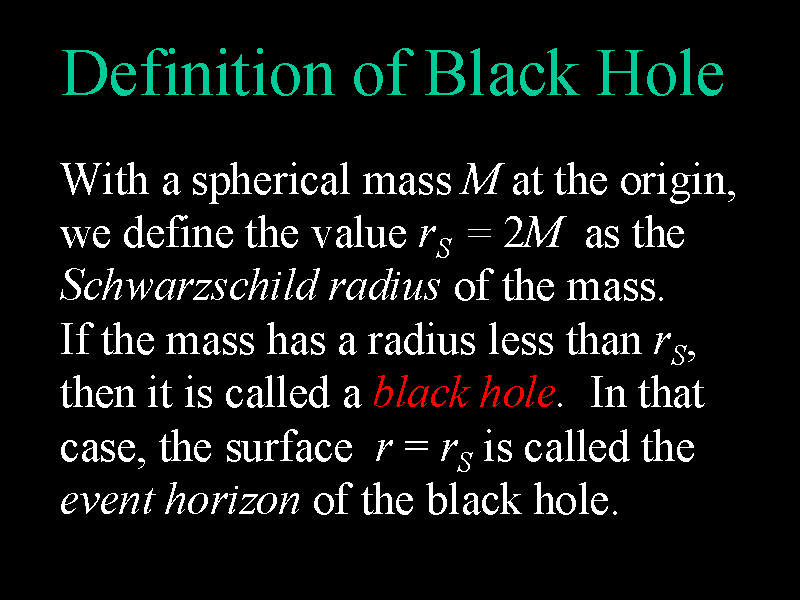In this setting of the spherical mass M, we define the value rS = 2M as the Schwarzschild radius of the mass. If the mass has a radius less than rS, then it is called a black hole. In that case, the surface r =rS is called the event horizon of the black hole.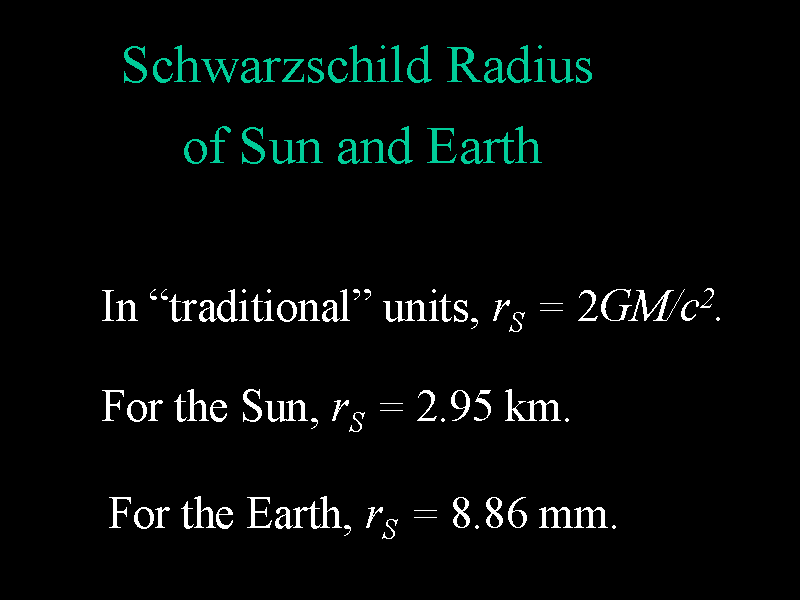In "traditional" units, the Schwarzschild radius of an object of mass M is rS = 2GM/c2. For the Sun, rS = 2.95 km and for Earth, rS = 8.86 mm. So if the Earth were compressed down to about the size of a pea, then it would be at the density of a black hole.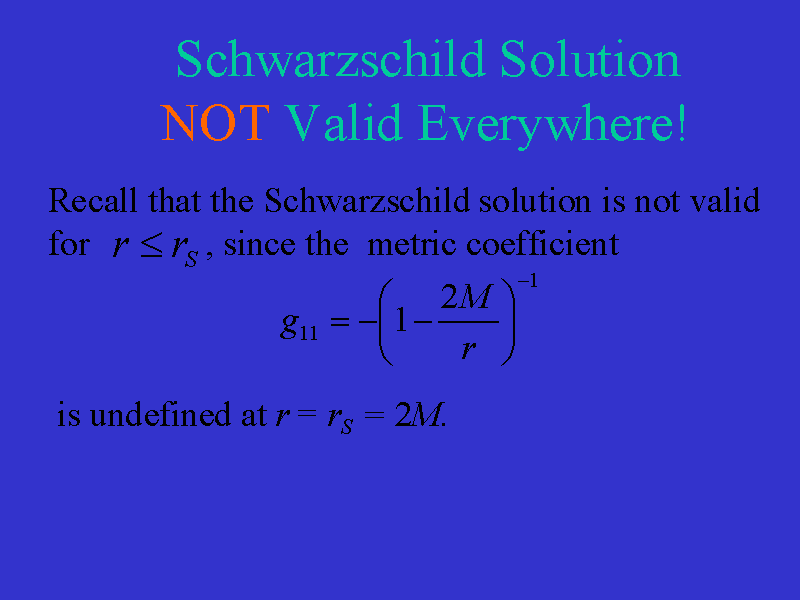We see that the Schwarzschild solution is not valid for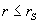since the metric coefficientis undefined at r = rS = 2M. We need a new set of coordinates to explore the behavior of the gravitational field of a black hole inside of the event horizon.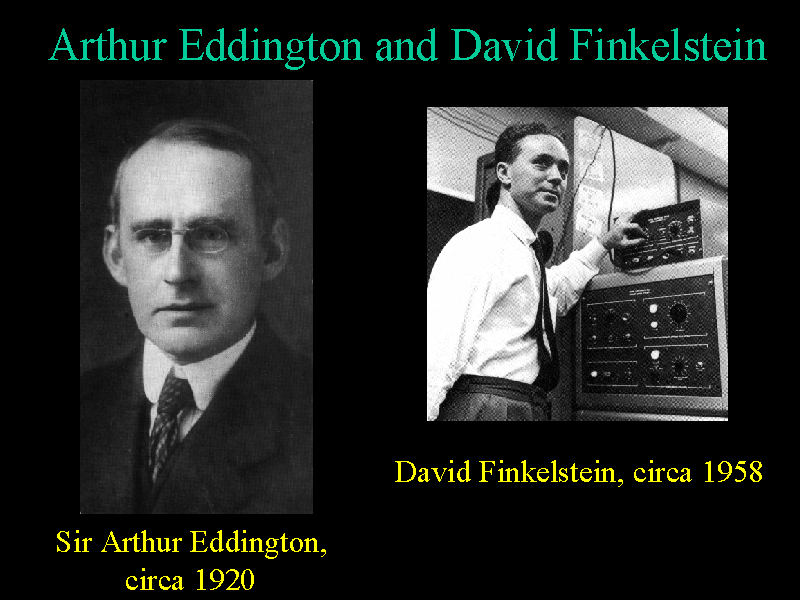Based on initial work by Arthur Eddington in 1924 and more detailed work by David Finkelstein in 1958, a system of coordinates was introduced which were valid for all r > rB. These are called the Eddington-Finklestein coordinates.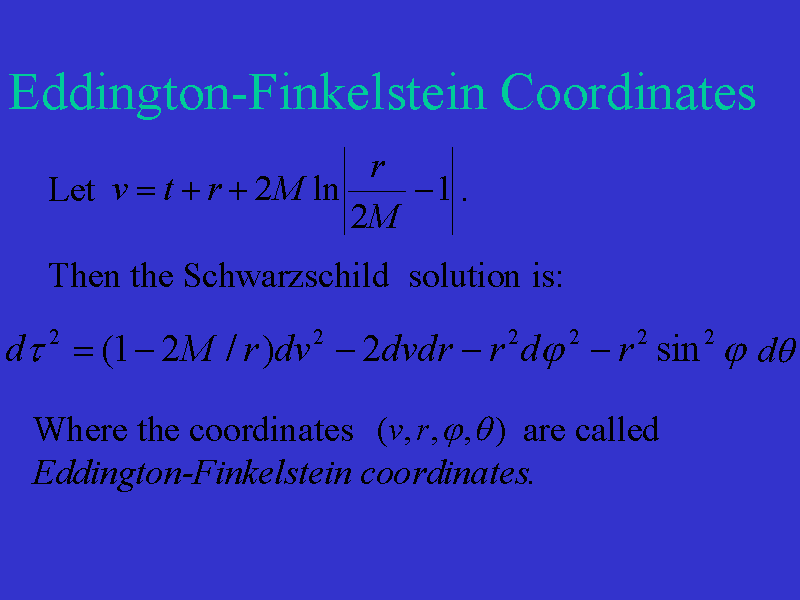We introduce the variable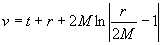. Then we can verify that the Schwarzschild solution becomes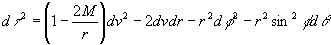This is the Schwarzschild solution in Eddington-Finkelstein coordinates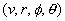. Notice that each of the metric coefficients is defined for all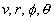and so we can use this version of the Schwarzschild solution to explore what happens for all nonzero r > rB.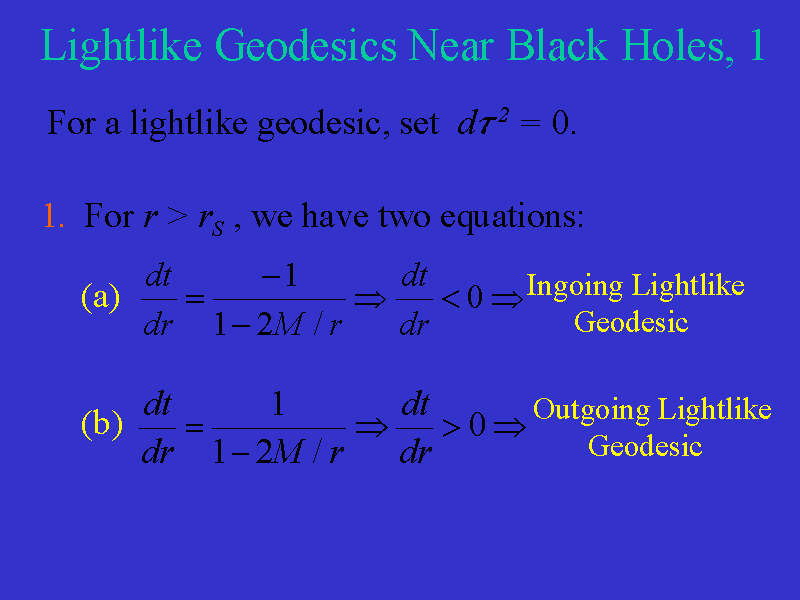Now let's consider what happens to photons emitted at a given distance from the center of a black hole. We ignore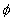and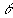and take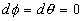. We want to study the radial path that photons follow. That is, we want to look at a radial lightlike geodesic. Therefore we set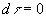and solve. First, consider the case r > rS. We are lead to two equations involving t and r. The first is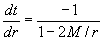Here, dt/dr is negative for r > rS and so as time t increases, distance from the origin r decreases. These represent the ingoing lightlike geodesics. The second equation in this case is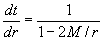Now, dt/dr is positive for r > rS and so as time increases, distance from the origin r increases. These represent the outgoing lightlike geodesics. Therefore a flash of light emitted at position r where r > rS will result in photons that go towards the black hole and photons that go away from the black hole.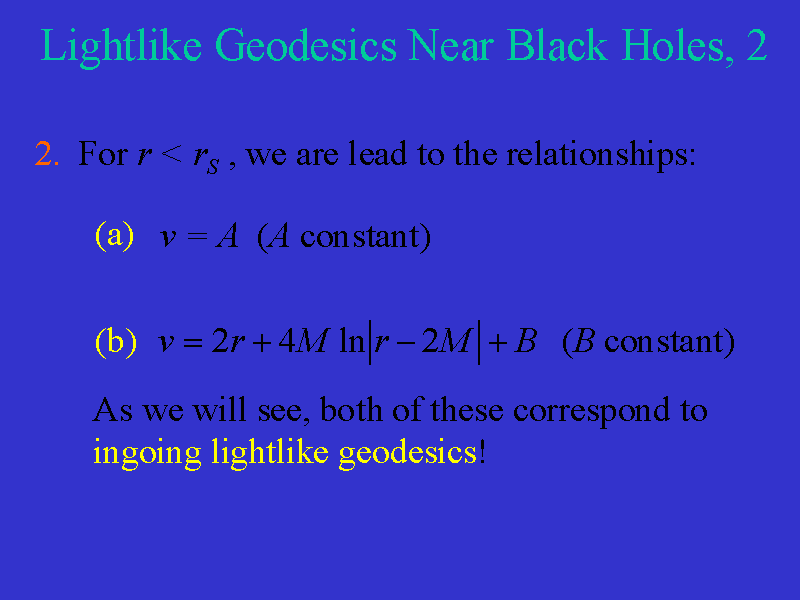Second, consider the case r < rS. We are lead to the relationships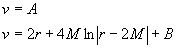where A and B are constant. As we will see, both of these correspond to ingoing lightlike geodesics! Let's look at a diagram that summarizes these results.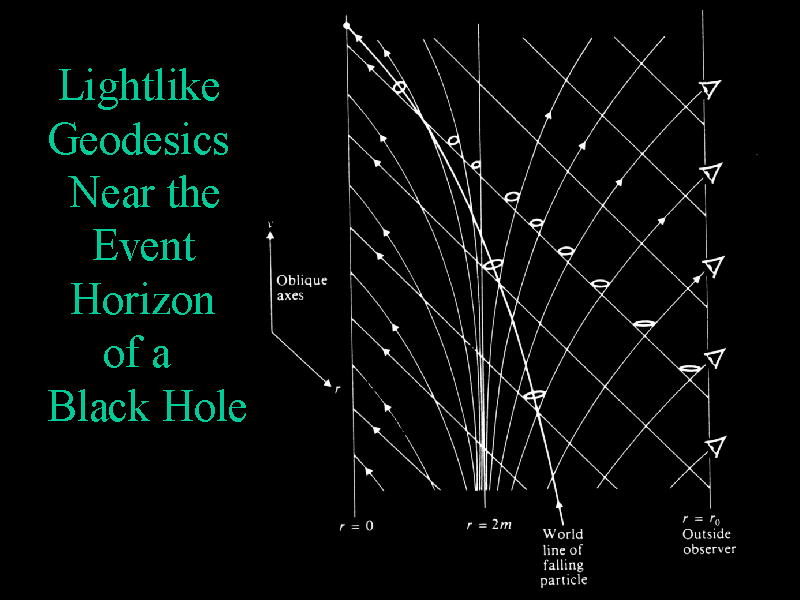Here we have a set of oblique axes r and v. Where the curves cross, we can imagine that a photon is emitted at these points and that the two curves represent the possible path of photons emitted at these points. Notice that a photon emitted towards the center of the black hole will travel to the center of the black hole (or at least to rB). A photon emitted away from the center of the black hole will escape the black hole if it is emitted at r > rS. However, such photons are "pulled" towards r = 0 if they are emitted at r < rS. Therefore, any light emitted at r < rS will not escape the black hole and therefore cannot be seen by an observer located at r > rS. Thus the name black hole. Similarly, an observer outside of the black hole cannot see any events that occur inand so (again) the sphere r = rS is called the event horizon of the black hole.

Notice the worldline of a particle which falls into the black hole. If it periodically releases a flash of light, then the outside observer will see the time between the flashes getting longer and longer. There will therefore be a gravitational redshift of photons emitted near r = rS with r > rS. Also notice that the outside observer will see the falling particle take longer and longer to reach r = rS. Therefore the outside observer sees this particle fall towards r = rS, but the particle appears to move slower and slower. On the other hand, if the falling particle looks out at the outside observer, it sees things happen very quickly for the outside observer. In fact, the falling particle will see the whole evolution of the universe to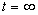. All this action, though, is compressed into the brief amount of time (in the particles frame) that it takes to fall to r = rS.

Notice that some of the radial lightlike geodesics have an asymptote at r = rS. This will result in lightcones tilting over towards the black hole as we approach r = rS.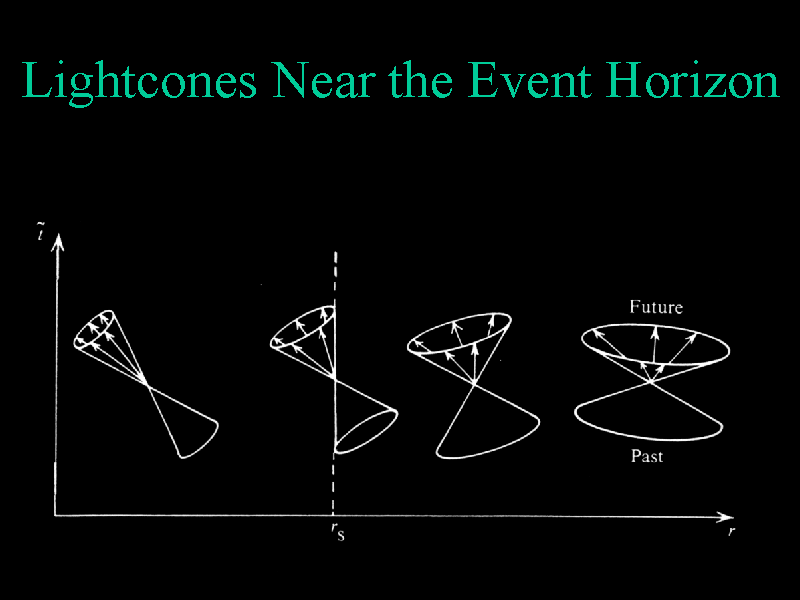Again, far from the black hole, light cones are as they appear in flat spacetime. For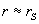and r > rS, light cones tilt over towards the black hole, but photons can still escape the black hole. At r = rS, photons are either trapped at r = rS (those emitted radially to the black hole) or are drawn into the black hole. For r < rS, all worldlines are directed towards r = 0. Therefore anything inside rS will be drawn to r = 0. All matter in a black hole is therefore concentrated at r = 0 in a singularity of infinite density.

Go to next section.

